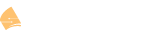# Hexadecimal to Decimal and Binary

This topic is to discuss the following lesson:

How to convert IPV6 to IPV4 address?

We can’t, there is no such thingIPv6 addresses are 128 bit and hexadecimal, IPv4 has 32 bit decimal addresses.

Typo: “The first nibble will be 8 + 4 = 12. The decimal value 7 is C in hexadecimal.”

Thanks Mathijs, it has been fixed.

Hi Rene,
“In the decimal system we count from 1-10, in the hexadecimal system we count from 1 – F”
should be “decimal 0-9, hexadecimal 0-F”Hi Georgi,

That is so true, especially for computersJust edited the post…thanks!

Rene

Hi Rene , I don’t understand how you convert nibbles binary to decimal,

Hello Emil

First of all let’s see the relationships between hex, and a nibble in binary. Each single hex digit corresponds to four bits in binary. But four bits in binary is considered a nibble. So a single hex digit corresponds to a nibble in binary. So when you are dealing with nibbles, it’s good to remember that you are dealing with four digits in binary, or one digit in hex.

If you know that, then it’s easy to convert from hex to decimal and decimal to hex. But when you convert from hex to binary, it’s usually easier to go from hex to decimal (which is trivial) to binary. The same when you go in the other direction, that is binary, to decimal to hex.

So let’s say you have the hex digit B. This corresponds to 11 in decimal. Which corresponds to 1011 (8+0+2+1=11) in binary, which is a nibble.

An example in the other direction could be 1110. This is 8+4+2=14 in decimal, which corresponds to E.

I hope this has been helpful!

Laz

Chaps,
I’ve been writing out the place value systems for binary, decimal and hexadecimal and have spotted a pattern that I can’t explain.

For instance…

In Binary - 2 ^ 3 = 1000
In Decimal - 10 ^ 3 = 1,000
In Hexadecimal - 16 ^ 3 = 1000

All results are 1000 even though this is expressed in the three different number systems so are different values.

The pattern repeats no matter what the next power of is:

In Binary - 2 ^ 7 = 10000000
In Decimal - 10 ^ 7 = 10,000,000
In Hexadecimal - 16 ^ 7 = 10000000

This is more of an observation that a question I suppose but if anyone can offer an explanation as to why this occurs then I’d appreciate it!

Cheers,

Gareth.

Hello Gareth

Just to clarify, I assume you mean the following:

• In binary: 2^3 means 10 X 10 X 10 = 1000
• In decimal: 10^3 means 10 X 10 X 10 = 1000
• in Hex: 16^3 means 10 X 10 X 10 = 1000

You’ll notice that the number 2 expressed in binary is 10, the number 10 in decimal is 10, and the number 16 expressed in hex is also 10. When you apply multiplication to the number 10 in any base system (binary, trinary, decimal, hex, or literally any base), you will always use the same methodology to multiply. Specifically, you will simply append zeros at the end.

This is a pattern that appears because of the fact that you are using the base value of each system (2 for binary, 10 for decimal, 16 for hex) in your calculation. This results in using the same symbols for all three numbers, which when multiplied results in the same symbols once again since the same process (multiplication) is applied in each case.

I hope this has been helpful!

Laz

Wow! And the number 1 in binary, decimal and hexadecimal is always 1 too… hmmmmm Sounds so obvious when you type it out (but it definitely still blows my mind somewhat).

I’m also trying to bend my mind around the fact that any number ^1 is always 1…

Thanks Laz - this is a great help.

Hi Gareth

I think you meant any number ^0 is always 1, right? Any number ^1 is simply itself. But it is quite interesting, this world of mathematics isn’t it!?!?!

Laz

1 LikeYep - any number to the ^0 is 1 - my bad!!!

1 Like